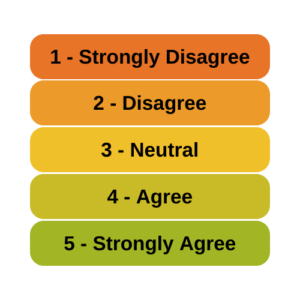# Factor Analysis

### Can Likert Scale Data ever be Continuous?

January 19th, 2023 by

A very common question is whether it is legitimate to use Likert scale data in parametric statistical procedures thatrequire interval data, such as Linear Regression, ANOVA, and Factor Analysis.

A typical Likert scale item has 5 to 11 points that indicate the degree of something. For example, it could measure agreement with a statement, such as 1=Strongly Disagree to 5=Strongly Agree. It can be a 1 to 5 scale, 0 to 10, etc. (more…)

### Correlated Errors in Confirmatory Factor Analysis

July 13th, 2022 by

Latent constructs, such as liberalism or conservatism, are theoretical and cannot be measured directly.But we can represent the latent construct by combining a set of questions on a scale, called indicators. We do this via factor analysis.

Often prior research has determined which indicators represent the latent construct. Prudent researchers will run a confirmatory factor analysis (CFA) to ensure the same indicators work in their sample.

### Member Training: Matrix Algebra for Data Analysts: A Primer

August 31st, 2021 by

If you’ve been doing data analysis for very long, you’ve certainly come across terms, concepts, and processes of matrix algebra.  Not just matrices, but:• Traces and determinants
• Eigenvalues and Eigenvectors
• Inverting and transposing
• Positive and negative definite

### Measurement Invariance and Multiple Group Analysis

October 23rd, 2020 byCreating a quality scale for a latent construct (a variable that cannot be directly measured with one variable) takes many steps. Structural Equation Modeling is set up well for this task.

One important step in creating scales is making sure the scale measures the latent construct equally well and the same way for different groups of individuals.

### How Big of a Sample Size do you need for Factor Analysis?

August 21st, 2020 by

Most of the time when we plan a sample size for a data set, it’s based on obtaining reasonable statistical power for a key analysis of that data set. These power calculations figure out how big a sample you need so that a certain width of a confidence interval or p-value will coincide with a scientifically meaningful effect size.

But that’s not the only issue in sample size, and not every statistical analysis uses p-values.

### Why Adding Values on a Scale Can Lead to Measurement Error

July 22nd, 2020 by

Whenever you use a multi-item scale to measure a construct, a key step is to create a score for each subject in the data set.This score is an estimate of the value of the latent construct (factor) the scale is measuring for each subject.  In fact, calculating this score is the final step of running a Confirmatory Factor Analysis.